A little EM review Coulombs Law Gausss Law

• Slides: 16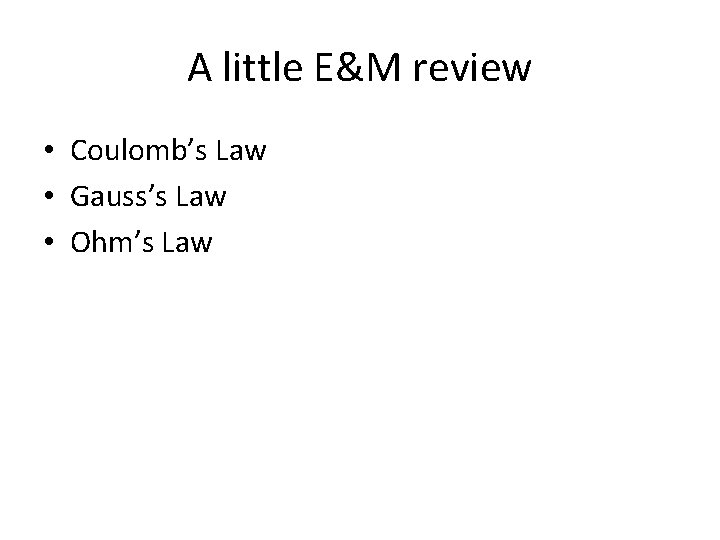A little E&M review • Coulomb’s Law • Gauss’s Law • Ohm’s Law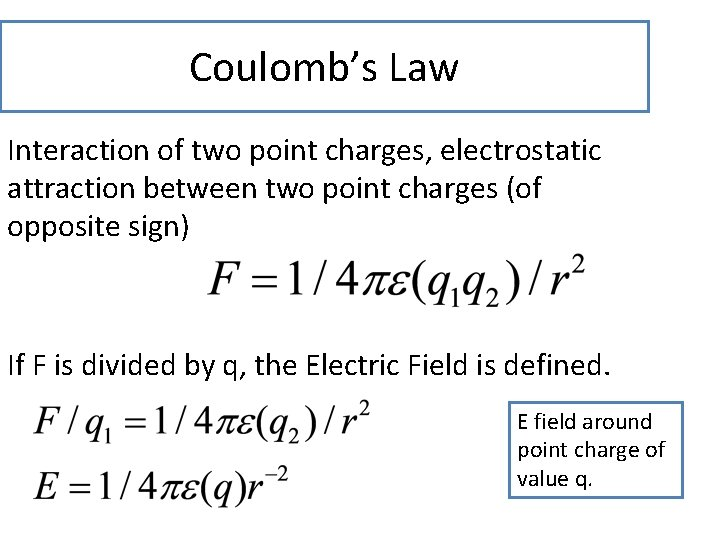Coulomb’s Law Interaction of two point charges, electrostatic attraction between two point charges (of opposite sign) If F is divided by q, the Electric Field is defined. E field around point charge of value q.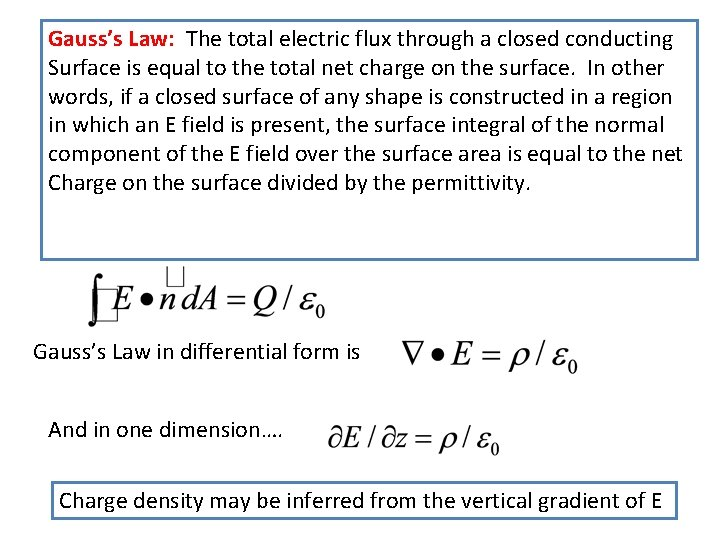Gauss’s Law: The total electric flux through a closed conducting Surface is equal to the total net charge on the surface. In other words, if a closed surface of any shape is constructed in a region in which an E field is present, the surface integral of the normal component of the E field over the surface area is equal to the net Charge on the surface divided by the permittivity. Gauss’s Law in differential form is And in one dimension…. Charge density may be inferred from the vertical gradient of EBalloon borne Electric Field Meter—EFM (Winn and Byerley (1975; QJRMS) Two 15 cm hollow copper spheres, separated by 2 cm. The spheres rotate at 2. 5 Hz. The in-cloud electric field causes opposite polarity of charge to develop on the two spheres which causes a sinusoidal signal at the spin rate. A mercury switch in the tube between the spheres switches on and off to determine which sphere is up. From that the sign of Ez can be determined. Charge density can then be derived by using Gauss’ Law in the vertical dimension.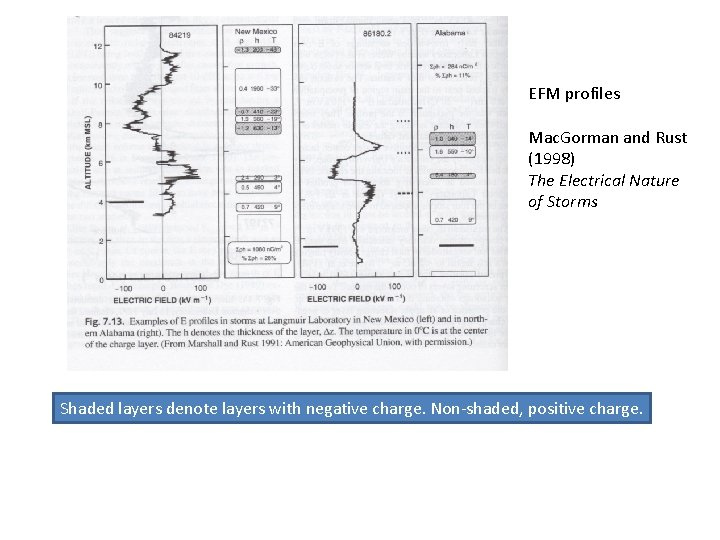EFM profiles Mac. Gorman and Rust (1998) The Electrical Nature of Storms Shaded layers denote layers with negative charge. Non-shaded, positive charge.Ohm’s Law J is the current density, coulombs/m 2 For the Earth’s atmosphere, J is 2. 7 x 10 -12 A m-2 (J constant with height in fair weather). This is the air earth current. Here the symbol is the conductivity with units of S/m, Siemens/meter, of Mhos Conductivity is a measure of the ease which charge moves through a given medium. Ohm’s Law may also be expressed in simple “circuit” form, V=IR where V is The voltage, I is the current and R is the resistance (Ohms) V I R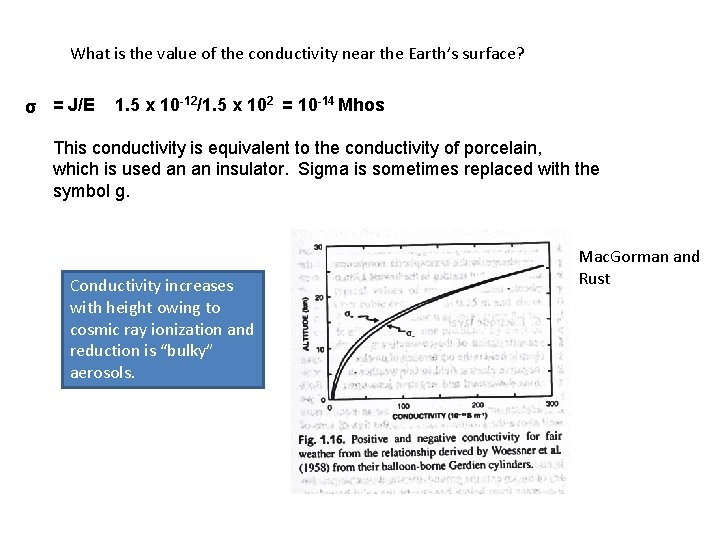What is the value of the conductivity near the Earth’s surface? σ = J/E 1. 5 x 10 -12/1. 5 x 102 = 10 -14 Mhos This conductivity is equivalent to the conductivity of porcelain, which is used an an insulator. Sigma is sometimes replaced with the symbol g. Conductivity increases with height owing to cosmic ray ionization and reduction is “bulky” aerosols. Mac. Gorman and RustVoltage difference is approximately 300 k. V Electrosphere, 50 -70 km altitude Inner circle is Earth, radius of 6370 km So we live in a giant capacitor. Earth’s surface is the lower plate and the electrosphere about 50 -70 km overhead is the upper plate. Upper plate is positive and the lower plate is negative. The voltage difference across the capacitor is about 300 k. V. What is the voltage difference across an individual 2 meters tall standing at the Earth’s surface?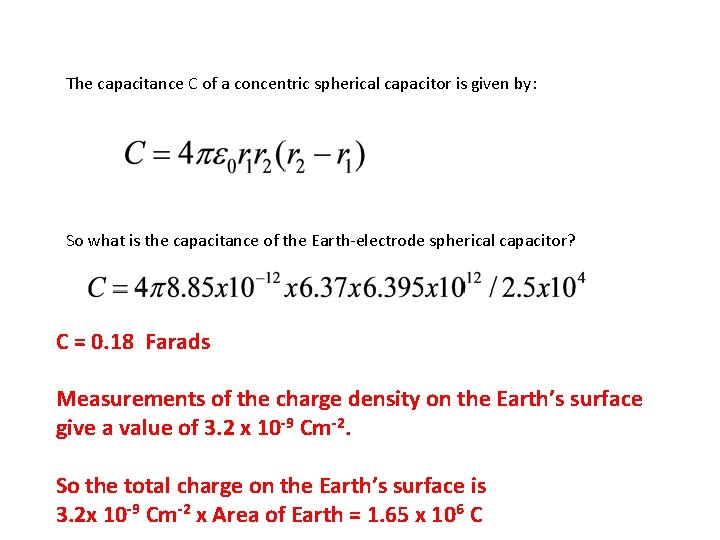The capacitance C of a concentric spherical capacitor is given by: So what is the capacitance of the Earth-electrode spherical capacitor? C = 0. 18 Farads Measurements of the charge density on the Earth’s surface give a value of 3. 2 x 10 -9 Cm-2. So the total charge on the Earth’s surface is 3. 2 x 10 -9 Cm-2 x Area of Earth = 1. 65 x 106 CWHAT IS THE ENERGY STORED IN THIS CAPACITOR? Energy =Q 2 C/2 Plugging in numbers gives 7 x 1012 Joules This is the energy of a million 12 V 100 Amp-hr automobile storage batteries.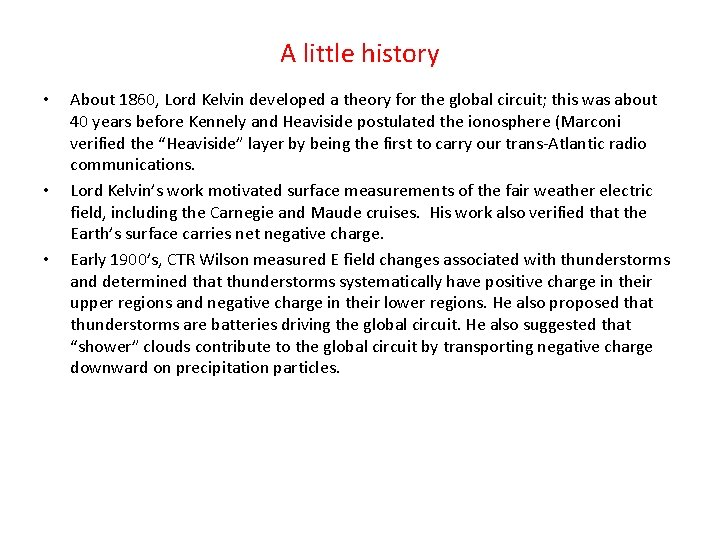A little history • • • About 1860, Lord Kelvin developed a theory for the global circuit; this was about 40 years before Kennely and Heaviside postulated the ionosphere (Marconi verified the “Heaviside” layer by being the first to carry our trans-Atlantic radio communications. Lord Kelvin’s work motivated surface measurements of the fair weather electric field, including the Carnegie and Maude cruises. His work also verified that the Earth’s surface carries net negative charge. Early 1900’s, CTR Wilson measured E field changes associated with thunderstorms and determined that thunderstorms systematically have positive charge in their upper regions and negative charge in their lower regions. He also proposed that thunderstorms are batteries driving the global circuit. He also suggested that “shower” clouds contribute to the global circuit by transporting negative charge downward on precipitation particles.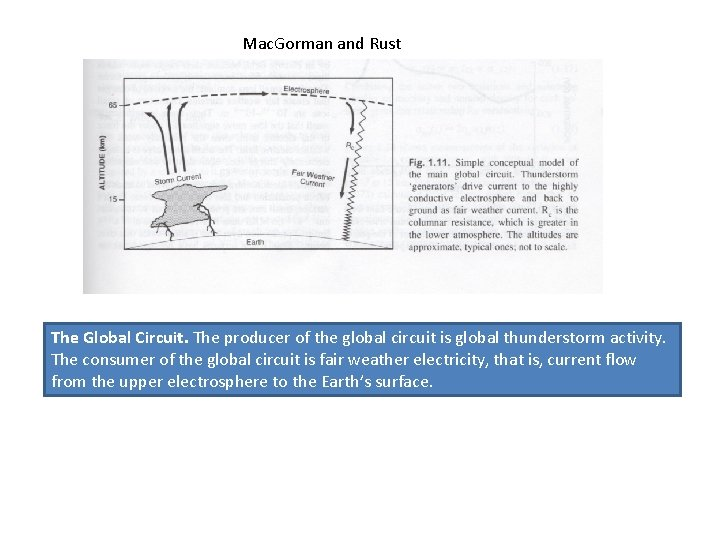Mac. Gorman and Rust The Global Circuit. The producer of the global circuit is global thunderstorm activity. The consumer of the global circuit is fair weather electricity, that is, current flow from the upper electrosphere to the Earth’s surface.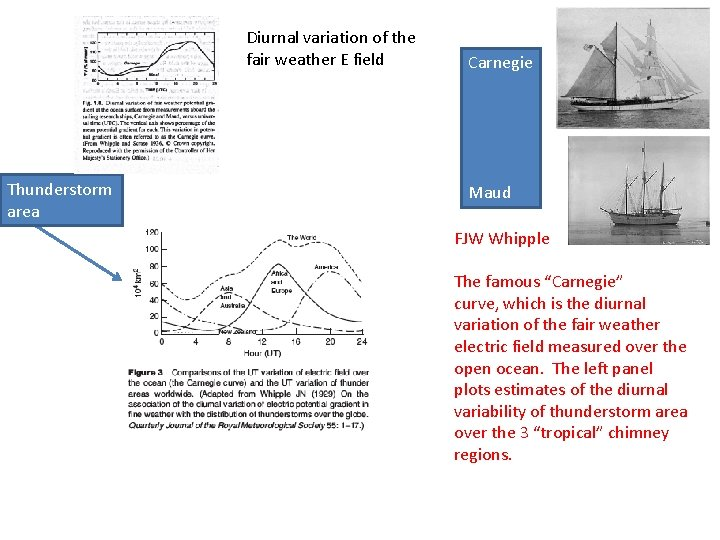Diurnal variation of the fair weather E field Thunderstorm area Carnegie Maud FJW Whipple The famous “Carnegie” curve, which is the diurnal variation of the fair weather electric field measured over the open ocean. The left panel plots estimates of the diurnal variability of thunderstorm area over the 3 “tropical” chimney regions.We have seen that the Earth’s concentric spherical capacitor is a “leaky” dielectric, That is the conductivity of the atmosphere is finite. Without the action of TRW’s that transfer net positive charge to the upper electrode and lower net negative charge to the lower electrode, the fair weather electric field would run down over time. We can estimate the e-folding time for the fair weather electric field. Here RC is the so-called time constant, the product of the atmosphere’s resistance and its capacitance.Combining the following equations…… e-folding time is about 15 minutes!!What is current flow in the global circuit? • We can find the current flowing in the global circuit quite easily. • What is the mean resistance of the atmosphere?